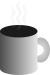#Coffee SpaceListen:

## Python LoopsPreview Image

Just a quick random one...

A friend recently proposed the following problem as part of their coursework (which I've changed to not give away the answers to others):

A sequence of numbers are defined as:

${z}_{1}=1$

${z}_{x+1}=13{z}_{x}+7$

You are to define a function `find_x(target)` that returns the smallest value of $x$ where ${z}_{x}$ is greater than or equal to the `target` value.

If implemented correctly, you should expect to see:

${z}_{1}=1$

${z}_{2}=13×1+7=20$

${z}_{3}=13×20+7=267$

${z}_{4}=13×267+7=3478$

Not too complicated - and I was really just helping with their debugging process as a new Python programmer.

### Initial Solution

Given how quickly something like this can be coded, I decided to whip something up so that I could test the correctness of their solution:

```0001 def find_x(target) :
0002   x = 1
0003   z_x = 1
0004   while z_x < target :
0005     z_x = (13 * z_x) + 7
0006     x += 1
0007   return x```

Testing:

```0008 print(find_x(1))
0009 print(find_x(10))
0010 print(find_x(100))
0011 print(find_x(1000))
0012 print(find_x(10000))
0013 print(find_x(100000))```

And the results of the program:

```0014 1
0015 2
0016 3
0017 4
0018 5
0019 6```

Passes the sniff test.

### More Interesting

So that is kind of boring, but probably of help for others. Let's dissect this a little and make it more interesting...

Firstly, what problems does this current version have? I.e. What traps are there for new players?

1. Negative values - We are not able to handle small values, we should gracefully fail. The sequence is undefined for $i\le 0$.
2. Calculation too large - We should be careful of overflows of large integer values during the calculation.
3. Slow for large values - This will be quite painful for really large values, but fortunately this won't be a problem for Python and its limitations for the maximum integer size.

For the first two, we can do something like (we'd likely pre-calculate `max_z_x`):

```0020 def find_x_v2(target) :
0021   max_z_x = (sys.maxint - 5) / 13
0022   assert(target >= 1)
0023   x = 1
0024   z_x = 1
0025   while z_x < target :
0026     assert(z_x < max_z_x)
0027     z_x = (13 * z_x) + 7
0028     x += 1
0029   return x```

And for testing:

```0030 print(find_x_v2(1))                     #  1
0031 print(find_x_v2(10))                    #  2
0032 print(find_x_v2(100))                   #  3
0033 print(find_x_v2(1000))                  #  4
0034 print(find_x_v2(10000))                 #  5
0035 print(find_x_v2(100000))                #  6
0036 print(find_x_v2(1000000))               #  7
0037 print(find_x_v2(10000000))              #  8
0038 print(find_x_v2(100000000))             #  9
0039 print(find_x_v2(1000000000))            # 10
0040 print(find_x_v2(10000000000))           # 11
0041 print(find_x_v2(100000000000))          # 12
0042 print(find_x_v2(1000000000000))         # 13
0043 print(find_x_v2(10000000000000))        # 14
0044 print(find_x_v2(100000000000000))       # 15
0045 print(find_x_v2(1000000000000000))      # 16
0046 print(find_x_v2(10000000000000000))     # 17
0047 print(find_x_v2(100000000000000000))    # 18
0048 print(find_x_v2(1000000000000000000))   # 19
0049 print(find_x_v2(10000000000000000000))  # 20
0050 print(find_x_v2(100000000000000000000)) # 21```

And as a result we get:

```0051 1
0052 2
0053 3
0054 4
0055 5
0056 6
0057 7
0058 8
0059 9
0060 9
0061 10
0062 11
0063 12
0064 13
0065 14
0066 15
0067 16
0068 17
0069 17
0070 Traceback (most recent call last):
0071   File "main.py", line 41, in <module>
0072     print(find_x_v2(10000000000000000000))  # 20
0073   File "main.py", line 17, in find_x_v2
0074     assert(z_x < max_z_x)
0075 AssertionError```

So it appears that Python correctly crashes for suitably large integer values.

### Even More Interesting

Start off with the following formula:

${z}_{x+1}=13{z}_{x}+7$

For the third case (skipping the second) we can write:

${z}_{x+1}=13\left(13{z}_{x-1}+7\right)+7$

${z}_{x+1}={13}^{2}{z}_{x-1}+\left(13×7\right)+7$

For the fourth case we can write:

${z}_{x+1}={13}^{2}\left(13{z}_{x-2}+7\right)+\left(13×7\right)+7$

${z}_{x+1}={13}^{3}{z}_{x-2}+\left({13}^{2}×7\right)+\left(13×7\right)+7$

There appears to be a pattern...

${z}_{x+1}={13}^{x}{z}_{1}+{13}^{x-1}7+...+{13}^{0}7$

For example:

${z}_{6}={13}^{5}+{13}^{4}7+{13}^{3}7+{13}^{2}7+{13}^{1}7+{13}^{0}7=587880$

Whilst it's annoying that we have this long term that expands, actually for the most part we can ignore it. The first term ${13}^{x-1}7$ is much larger than all the summed terms down to ${13}^{0}7$. For the search we can therefore simplify to:

${z}_{x+1}={13}^{x}+{13}^{x-1}7$

We are trying to find something around the target, $t$, so now we search for:

$t={13}^{x}+{13}^{x-1}7$

This can be rewritten as:

$13t=20×{13}^{x}$

$\frac{13t}{20}={13}^{x}$

$lo{g}_{13}\left(\frac{13t}{20}\right)=x$

And now we have something for approximating the location of some positive value of $x$ given some target $t$.

I'm not going to go to the effort of programming this (because I don't care so much), but it's interesting to see that it's possible to find an approximate solution very quickly, without being required to do a large loop. In theory you can now find arbitrary values by performing just a 'few' calculations either side of the predicted value 1.

1. I would need to think about exactly a search strategy.